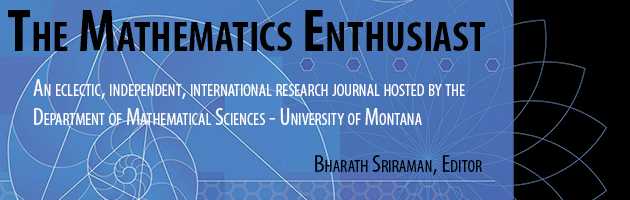•
•#### Article Title

Number series and computer

16

1-3

#### Abstract

This article describes the discovery and the subsequent proof of a hypothesis concerning harmonic series. The whole situation happened directly in the course of the process of a maths lesson. The formulation of the hypothesis was supported by the computer. The hypothesis concerns an interesting connection between harmonic series and the Euler's number 𝑒. Let 𝐻𝑛 denote the 𝑛’th partial sum of the harmonic series. Let’s notice the sums 𝐻1, 𝐻4, 𝐻11, . . ., where the partial sum reaches 1, 2, 3, . . . for the first time. Let’s mark the relevant indexes 1, 4, 11, . . . as 𝑝1, 𝑝2, 𝑝3, . . .. So 𝑝𝑛 is the index of such a partial sum for which the following is true: 𝐻P𝑛−1 < n, 𝐻P𝑛 > n. The hypothesis lim Pn+1/Pn = e has been proved, in an elementary way, in the article.

253

262

COinS